﻿ New Discoveries and the Necessity of Reconsidering the Perspectives on Newton's Second LawJournal of Nuclear and Particle Physics

p-ISSN: 2167-6895    e-ISSN: 2167-6909

2012;  2(3): 31-35

doi:10.5923/j.jnpp.20120203.02

### New Discoveries and the Necessity of Reconsidering the Perspectives on Newton's Second Law

H. Javadi1, F. Forouzbakhsh2, A. Jahanshir3, H. Pour Imani4

1the Faculty of Science at Azad Islamic University, Science and Research Campuses, 11365-4435, Tehran, Iran

2Office of the Vice-chancellor for Research and Technology, University of Tehran, 19466-14947, Tehran, Iran

3Department of theoretical physics, Faculty of physics, FKN University, Almaty, 92 Tolebi St., Kazakhstan

4Physics Research Department Northwest Arkansas, University of Arkansas, Arkansas, 72701, USA

Correspondence to: H. Javadi, the Faculty of Science at Azad Islamic University, Science and Research Campuses, 11365-4435, Tehran, Iran.
 Email: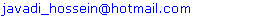Abstract

According to the mainstream physicists the observation and record of the motion of neutrinos at ultra relativistic speeds apparently seem to violate relativity postulate and to violate the speed of light as a universal constant. The Einstein mass-energy equation E=mc2, the relativistic mass, relativistic form of Newton’s second law, the fundamental principles and equations of modern physics by generalizing this anomaly will be challenged. Some other observations and results make possible the speeds higher than speed of light which is not rather incredible and it will not be impossible; it only requires a change in today’s attitudes towards relativity and quantum mechanics. Thus this paper, from a new approach, turns out to merge the fundamental principles of quantum physics, relativity and classical mechanics through a new definition of quiescent state of particles like photon, and attempts to present the reasons and the possibilities of the existence of the superluminal speeds. At the beginning of the 20th century, Newton’s second law was corrected considering the limit speed c and the relativistic mass. At that time there has not been a clear understanding of the subatomic particles and basically there was little research in high energy physics, if we ignore the zero rest mass of photon, much better and more real physical phenomena may be investigated.The speed of the created particles is a function of the internal interaction and the mechanism of creation of subatomic particles, and the external forces that are exerted on them.

Keywords: Sub-Quantum Energy, New Structure Of Matter, Ultra Relativistic Speed, Linear Velocity and Momentum

Cite this paper: H. Javadi, F. Forouzbakhsh, A. Jahanshir, H. Pour Imani, New Discoveries and the Necessity of Reconsidering the Perspectives on Newton's Second Law, Journal of Nuclear and Particle Physics, Vol. 2 No. 3, 2012, pp. 31-35. doi: 10.5923/j.jnpp.20120203.02.

### 1. Introduction

Recently the physicists of CERN Lab. recognized that neutrinos move at speeds faster than speed of light [1, 2, 3]. It seems that one of the physics postulates has been challenged. Experimental Proof of particles’ motions at superluminal speeds challenges the relativistic mass, the relativistic relations and Newton’s second law. In this paper, it has been attempted to scrutinize the interface between relativity and quantum mechanics through a novel approach to the established physical events. Universal constancy of the speed of light undergoes the question whether the limit on the light speed originates from a natural event or not?
Regarding the phenomena of creation and decay of electron-positron pair , why do the related photons move at constant speed, but we could change the speed of matter and antimatter? What is the unique characteristic of matter which is convertible to photons that move with constant speed c (speed of light)? The idea that object/particle could not travel at superluminal speeds, originates from the structure of matter and the mechanism of interaction between field and mass; that with presenting a postulate we could generalize the constancy of speed from energy to mass. By gravitational blue shift, the energy of photon and consequently its frequency will increase. What is the mechanism of increasing in the photon energy that causes increase in its frequency growth?
Emphasizing on these phenomena and presenting the relation between photon’s energy and frequency, this paper is to draw attention on the importance of constancy of speed in relation to the mass structure which will be scrutinized in reviewing Newton’s second law.
In this view, the three major theories of classical mechanics, relativity theory and quantum mechanics relying on the law of conservation of mass - energy and momentum conservation, in the form of Newton's second law are combined. And thus the real and true definition of the photon mass will be presented. Such approach to the particles and forces are accenting on the mass as the intrinsic properties of elementary particles that we are indebted to classical mechanics. The energy has the mass is obtained from relativity and the mass variations in order to catch energy is acceptable and reviewable. The results of this study have been proposed in CPH-Theory , due to that many uncertainties and unanswered questions in modern physics, cosmology, before the big bang and its reasons

### 2. Sub-Quantum Energy

According to the principles of modern physics, Sub-quantum energy (SQE)  is preferred and defined in a way that it could be generalized and by using it, explained quantum and relativistic phenomena.
Definition: Sub-quantum energy is the least energy that is defined as below: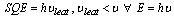(1)
Where ν is detectable.
Relation (1) shows that SQE in terms of energy is the smallest photon in nature. Every other photon consists of some SQE.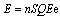(2)
Where n is an integer.
For two photons with energies E1 and E2 we have: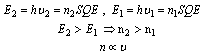(3)
Where n1 and n2 are integer.
With increasing in a photon’s energy, its frequency also increases. Thus there should be a logical explanation between energy increases and frequency increases. Therefore, based on SQE definition and relation (2) could relate the relation between photon’s energy and frequency and the interaction between SQE’s in photon’s structure, i.e. with the increase number of SQE’s in photons, the interaction between SQE’s in photons will increase and the frequency that originates from the interaction between SQE’s will increases.
Note: Although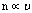, this proportion does not necessarily represent an equation, but simply represents the physical fact that frequency has direct relation with the number and interaction of SQE’s in photon. Besides the relation between SQE’s and,could conclude that the SQE linear speed in vacuum relative to the inertial frames of reference, is actually the speed of light c. Since SQE in photon’s structure has a linear speed equal to c and also it has nonlinear motions, the real speed of SQE is when all SQE nonlinear motions turn into linear motions and it only takes linear motion. In other words the limit speed of SQE is VSQE which is faster than light speed c, i.e.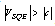.
Consider that in special relativity the light speed is constant, and in general relativity besides increasing of photon frequency while falling in a gravitational field, its speed also increases; that we could take it as a proof of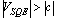.

### 3. Sub-Quantum Energy Principle

One SQE is a very small energy with nonzero mass (mSQE) moves atrelative to inertial reference frame and in every interaction between SQE’s with other particles or fields the speed value of SQE remains constant; as in every physical condition we have: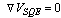(4)
in all inertial reference frames and any space SQE principle shows that in every condition the speed value of SQE remains constant and only the linear speed of SQE converts to nonlinear speed or vice versa.

### 4. Photon Mass

Considering the definition of SQE, every photon consist of some SQE, if we ignore the zero rest mass of photon, much better and more real physical phenomena may be investigated. Thus, a photon with energy E has a mass m=E/c2 and a linear momentum p=mc. In other words, a photon is a part of matter and has nonzero mass before creation that after converting to photon carries the same mass that had in the matter and after absorption by a particle (e.g. an electron), the mass of photon is added to the mass of the particle.

### 5. Gravitational Blue Shift

When a photon with energy E1 and frequency ν1 is falling in a gravitational field, after moving the distance h it takes the energy E2 and the frequency ν2. Considering the relation (2) could write as below: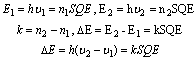The energy of photon while falling in a gravitational field, increases as ΔΕ and consequently causes to increase in the frequency of photon as Δν=ν2 -ν1. The change in frequency only originates from increasing in ΔΕ. The more ΔΕ increases, the more increase occurs in Δν, i.e. the number of SQE’s that entered the structure of photon is more.

### 6. Pair Creation and Decay

A photon with energy E in collision with a nuclei converts to an electron-positron pair. Considering the definition of photon and relation (2) we have: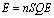From SQE principle it is concluded that the SQE exists in photon move at VSQE, some of which are in the form of linear motion with the value of c and the rest of them is in the form of nonlinear motions. When photon collides with a nucleus, the forces exerted to SQE’s cause the conversion of some other linear motions of SQE’s to nonlinear motions, and electron-positron pair is created. In the inverse procedure, with absorbing each other, electron and positron decay and convert to energy. While electron-positron pair decays, the force that electron and positron exert on each other causes the conversion of some of electron and positron nonlinear motion to linear motion, and thus the created photons move at c considering relation (4) could write: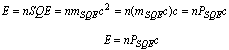(5)
In order to create electron-positron pair could write: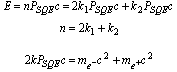The linear momentum of electron and positron after creation is as: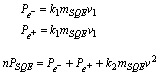(6)
And k2mSQEv2 is the pure momentum that transfers from photon to nuclei. The effect of nuclei reaction on the rest of existing SQE’s within the photon is the change of their momentum from linear to nonlinear. Even if k2=0, while colliding, the stroke from the nuclei to SQE’s of photons cause the conversion of linear momentum of SQE’s to nonlinear momentum and the reaction of SQE’s on nuclei is that nuclei is recoiled. Thus: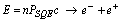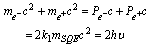(7)
In pair decay, because of the force that electron and positron exert on each other, some of the SQE’s nonlinear speeds which constitute the structure of these two particles convert to linear speed and two photons are created and move at c. Thus, the constancy of the speed of light does not rise from a natural event, but it is axiomatic and that is because matter (condensed energy) also consists of sub-quantum energy that moves at constant and limit speed value of VSQE When the binding forces among some of SQE’s and matter lose their effect (e.g., chemical reactions, combustion, nuclear explosion etc.) some of SQE is released in form of energy and move at linear speed c. If the released energy, even when it is a portion of matter and before converting to energy, move at constant speed, logically the constancy the speed of light would be possible.

### 7. Boucher Experiment

In Boucher1 experiment if we consider the initial mass of electron m0 and the output electron m, we have: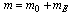mE is the gained mass of energy by electron in acceleration (exerting external force). Considering the relation (2) we have: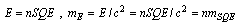Thus;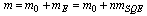In reality is that in Boucherer experiment, an electron in acceleration gains energy and after exiting from the accelerator tunnel, because of collision with another particle or because of passing through a field that gives it negative acceleration, it loses the energy and in terms of mass it returns back to its former state (the inverse form of Compton effect ). One could always use this experiment to prove relativity mass, but could not explain the real interaction between force and mass with relativistic mass.

### 8. Reconsider Newton’s Second Law

Newton’s second laws in classical mechanics which the mass was given as constant value and it was defined as follows: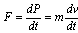(8)
By considering relativity and the speed limit of light, in order to propose the speed limit, the relation (8) was modified. Thus the relativistic mass and the interaction between force and mass were presented as follows: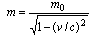(9)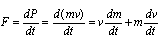(10)
Due to the relations (9) and (10), no force could extend/transmit the object/particle’s speed faster than the speed of light. It would be acceptable that the external force action is limited, but the reason is not the mass variations rather as it accented above, the reason of the speed limit should be sought in the structure of matter. According to the definition of the photon and SQE, Newton's second law could be reconsidered.
By assuming an electron at moment t1, with the mass m and the speed v1 along an axis in the field (on an inertial frame in the gravitational or electrical field), under the force F and at the moment t2, so its speed becomes v. Electron takes energy dE in the interval dt=t2-t1. According to relation (5) we have:
At the moment t1: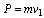Within the time dt=t2-t1, the electron gains energy as dE
At this time the electron momentum changes to the following value: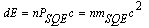At the moment t2 one could write: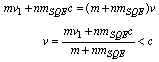(11)
Because of: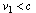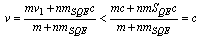As v1 , so always v.
Here one could correlate increased mass to the gain of energy in Newton's second law, i.e.: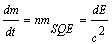And Newton's second law could be rewritten as below: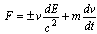(12)
The ± sign in relation (12) has been marked on the increasing and decreasing state of energy (collinear or non-collinear directional variations in force and speed). The relativistic mass uses in high energies just for showing the speed limit in quantum equations while for well-known subatomic particles always v

### 9. Conclusions

At the beginning of the 20th century, Newton’s second law was corrected considering the limit speed c and the relativistic mass. At that time there has not been a clear understanding of the subatomic particles and basically there was little research in high energy physics. Moreover, the approach of relativity toward the physical phenomena is hyper structural and explains the observations of the observer while there is little consideration to the intrinsic entity of the phenomena. However, in this paper, through various arguments and investigation of some physical phenomena, it has been attempted to show the necessity of reviewing Newton’s second law. Today Physics literature faces numerous problems and questions that without considering the internal structure of the particles, they would remain unanswered. Moreover, the classical definition of energy that defines energy as the ability to do work, could not explain the interaction among the particle in high energies. The true understanding of physical entity of energy and the structure of photon, enable us to understand the structure of matter. Moreover, Newton’s second law is the only relation that shows the interaction between force and matter. This equation has the sufficient efficiency to explain and investigate physical phenomena, when it would be formulated based on the natural reality of matter and the effect of force on the matter. The reality is that the external force, no way and under any physical condition, could not change the speed value and it only could convert the linear motion of the constituting particles of matter and energy to the nonlinear motion and vice versa. Moreover, one could explain the expansion of the universe better and more real through reviewing Newton’s second law.

### ACKNOWLEDGEMENTS

The authors would like to express their sincere gratitude and appreciation to Mr. Masoud Sanati, for his fruitful collaboration.

### Note

1. Alfred Heinrich Boucher (1863-1927) who is known for his experiments on relativistic mass.

### References

  The OPERA Collaboraton: T. Adam, N. Agafonova, at al., “Measurement of the neutrino velocity with the OPERA detector in the CNGS beam”, arXiv:1109.4897v2 [hep-ex], 2011.  G.Andrew Cohen, L. Sheldon Glashow, “New Constraints on Neutrino Velocities”, arXiv: 1109.6562v1 [hep-ph], 2011.  D. Fargion, D. D'Armiento, “Inconsistence of super-luminal Opera neutrino speed with SN1987A neutrinos burst and with flavor neutrino mixing”, arXiv:1109.5368v5 [astro-ph.HE], 2011.  H. Nitta, M. Kh. Khokonov, at. al., “Eletctron-Positron Pair Production By Photons In Nonuniform Strong Fields”, Phys. Rev. Lett., 93(18), 1804071-7, 2004.  H. Javadi, “Unified Mass and Energy or Creative Particles of Higgs or CPH Theory”, Scientific-Technical Journal of Islamic Azad University, South Branch, vol. 1, pp. 15- 17, 1991.  H. Javadi, F. Forouzbakhsh, (2007), “Zero point Energy and Dirac Equation, SQE or Tiny Energy or Minute electromagnetic Energy”, the general science journal, [Online] available:http://www.gsjournal.net/Science-Journals/Essays/View/950  T. Padmanabhan, “Inverse Compton Scattering – Revisited”, J. Astrophys. Astr., vol.18, pp.87-90, 1997.